#The 5th grade math games on this website focus on several important topics such as adding, subtracting, multiplying, and dividing decimals and fractions, investigating algebraic expressions, computing area and volume of simple geometric figures, understanding the meaning of congruent geometric shapes and the relationship of the circumference of a circle to its diameter, and using percentages and circle graphs to interpret statistical data.

Sharpen your math skills by playing these fun games!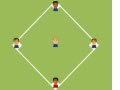Adding Decimals Baseball Game

This is a fun baseball game that can be played alone, in pairs, or in two teams. To have a chance to hit a homerun, you must answer each math problem correctly.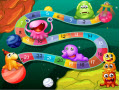Decimals Board Game (New)

Practice adding, subtracting, multiplying, and dividing decimals in this challenging online math board game.Compare Decimals Math Racing Game

Compare decimals in order to finish the race first in this wonderful Math racing game. If you like hairpin turns and bumper-to-bumper fast-paced action, try this game.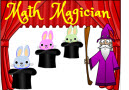Math Magician Equivalent Fractions Game

Match the equivalent fractions with the correct solutions on the hats in this fun Math Magician Equivalent Fractions Game.Adding and Subtracting Fractions Board Game (New)

Play this cool online board game about fractions, and be the first student in your class to cross the finish line. For each correct answer, you will be able to roll a virtual die and advance on the board towards victory.Round Decimals Math Racing Game

Round decimals in order to finish the race first in this cool Math racing game. Watch out for the hairpin turns and bumper-to-bumper fast-paced action by working quickly but carefully.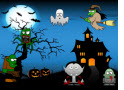5th Grade Halloween Math Game (New)

Play this fun, online Halloween math game and practice your math skills to destroy a lot of monsters. For each correct answer, you will enter a bonus round where you can earn points by smashing monsters. The math problems are about ordering multi-digit numbers from least to greatest and greatest to least.Division With Remainder Game

In this multi-player soccer game, kids will solve different division with remainder problems.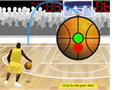Adding Decimals Basketball Game

Students can play this online math basketball game to practice their math skills in adding decimals.Math Magician Simplest Form Game

Quickly drag and drop the fractions on the bunnies to the correct simplest form of the fractions on the hats in this fun Math Magician Simplest Form Game.Estimate Quotients Halloween Math Game (New)

This enjoyable Estimate Quotients Halloween Math Game lets you get plenty of practice estimating quotients by using compatible numbers. Test your math skills and have fun destroying spooky monsters.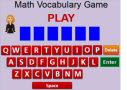5th Grade Math Vocabulary Game (New)

Do you know the key math vocabulary words that students should learn by the end of fifth grade? Play this fun and interactive math game to test your math vocabulary knowledge.Multiplication Board Game (New)

Play this fun multiplication board game and be the first one to get to the finish line. For each correct answer, you will be able to roll the die and advance on the board game towards the finish line.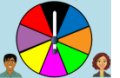Prime Factorization Game

In this fun spinner game, 5th grade students will find the prime factorization of two and three-digit composite numbers.Basketball Math - Multiplying Decimals

This is a fast-paced basketball game about multiplying decimals. Students can play it alone, with another friend, or even in teams.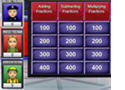Decimal Jeopardy Game

This game is a fun way to review basic operations with decimals. It has a single-player mode as well as a multi-player feature. Students can play this game at home or at school as a review activity.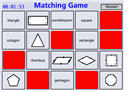2D Shapes Game (Concentration)

In this game students click on two cards to match the figure of a two-dimensional shape with its name. If there is a match, the problems remain on the page; if not, the cards are turned over.Place Value Word Search

This is an online word search game about place value vocabulary words.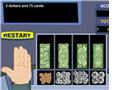Money Game

In this game, students will solve word problems about money. The game has a virtual cash register that students will use to solve the problems.Factors Millionaire Game

Students can play this game to assess their knowledge about factors and divisibility. It can be used as a review activity in the classroom or at home.Factors and Multiples - Jeopardy Game

The questions in this jeopardy game focus on important concepts such as factors, multiples, prime factorization, GCF, and LCM.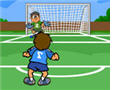Soccer Math Game - Simplifying Fractions

In this game 5th grade students will practice reducing fractions to the simplest form.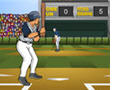Baseball Math Game - Simplifying Fractions

This is another game about simplifying fractions. Students will have to first hit a homerun to be able to see and answer a math problem.Fractions Jeopardy Game

This online jeopardy game is is a fun way to review the four operations with fractions: addition, subtraction, multiplication, and division.Football Math Game - Adding Fractions

In this game, students will try to pass the ball to the receiver. For each good pass, they will get to answer a math problem about adding fractions. For each correct answer they will earn 7 points, but each wrong answer will cost them 3 points.Changing Fractions and Decimals to Percents

This millionaire game can be played in one or two teams. Students will practice changing decimals and fractions to percents.Fractions, Decimals, Percents

In this online jeopardy game students will practice converting fractions to decimals and percents and vice-versa.Multiplying Fractions Game

Get ready to have some fun multiplying fractions when you play this exciting soccer math game.Multiplying Fractions Millionaire Game

In this online millionaire game, 5th grade students will practice multiplying fractions while earning lots of points.Algebraic Expressions Millionaire Game

This millionaire game can be played alone or in two teams. Students will have to identify the correct mathematical expressions that model given word expressions.

Return from the 5th Grade Math Games page to the Elementary Math Games page or to the Math Play homepage.